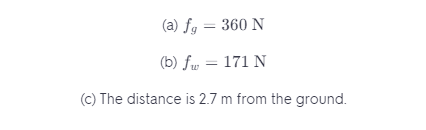# What Is The Maximum Frictional Force That The Ground Can Exert On The Ladder At Its Lower End?

We thoroughly check each answer to a question to provide you with the most correct answers. Found a mistake? Let us know about it through the REPORT button at the bottom of the page.

A uniform ladder 5.0 m long rests against a frictionless, vertical wall with its lower end 3.0 m from the wall. The ladder weighs 160 N. The coefficient of static friction between the foot of the ladder and the ground is 0.40. A man weighing 740 N climbs slowly up the ladder. Start by drawing a free-body diagram of the ladder.

(a) What is the maximum friction force that the ground can exert on the ladder at its lower end?

(b) What is the actual friction force when the man has climbed 1.0 m along the ladder?

(c) How far along the ladder can the man climb before the ladder starts to slip?

Contents

A)

The ladder is an equilibrium state. As shown in the figure below, the ground exerts normal force \$N_2\$ and the wall exerts a normal force N1. The friction force by the ground is given by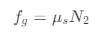The friction force by the wall is zero as it is frictionless, so the equilibrium of the ladder occurs due to the balance between the normal force N2 and the weight of the ladder Wl and the man Wm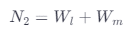Now, plug the values for Wl and Wm to get N2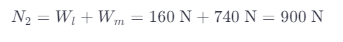Now, we plug the values for N2 and Us – 0.40 into equation (l) to get Fg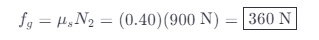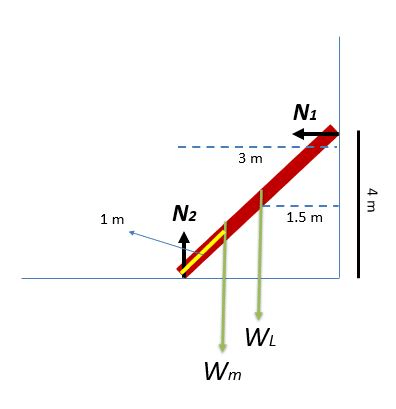B)

We need to find N2, there is a torque applied in the ladder, the summation of this torque is zero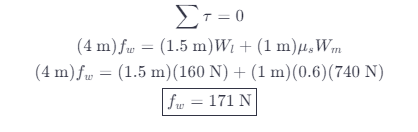C)

Let us consider the distance where the ladder starts to slip is X. At height 4 m, the maximum normal force of the wall N1 is the same for the ground N2 – 360 N. So, the torque before the ladder starts to slip is zero.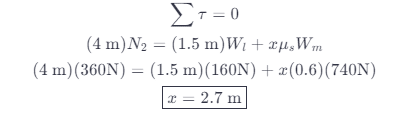## Result